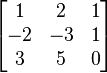## Rank of 3 * 3 Matrix Calculator

#### Enter values

Rank:

The rank of a matrix A is The maximum number of linearly independent rows in a matrix A (the row rank) or the maximum number of linarly independent columns in A (the column rank).

The 3*3 matrix is the most common matrix, You input the number elements and click "Calculate" get the Rank(A).

### Examplesthe first two rows are linearly independent, so the rank is at least 2, but all three rows are linearly dependent so the rank must be less than 3. The matrix has rank 2.

Thinkcalculator.com provides you helpful and handy calculator resources.